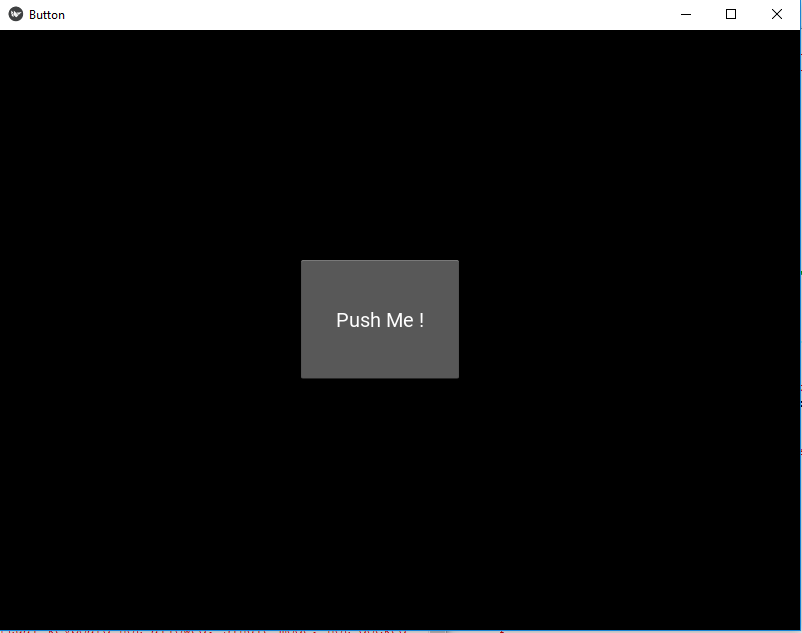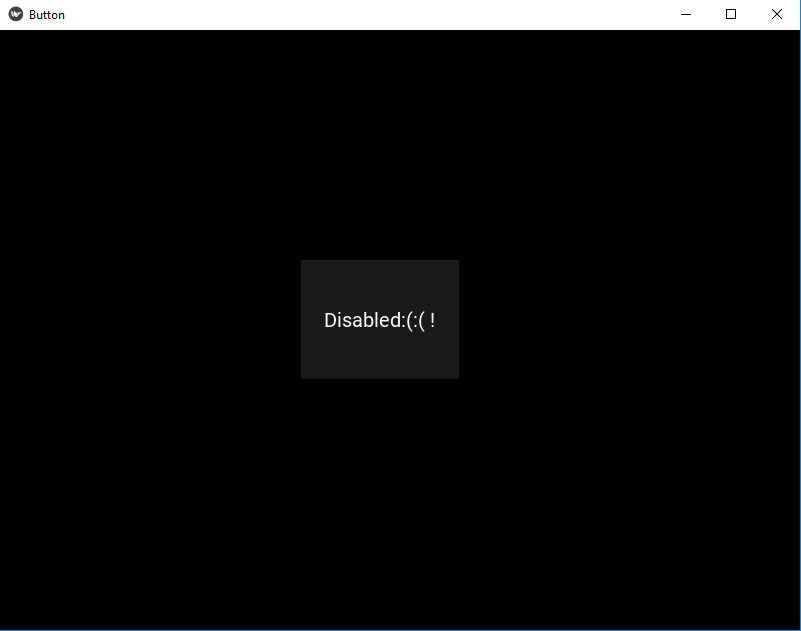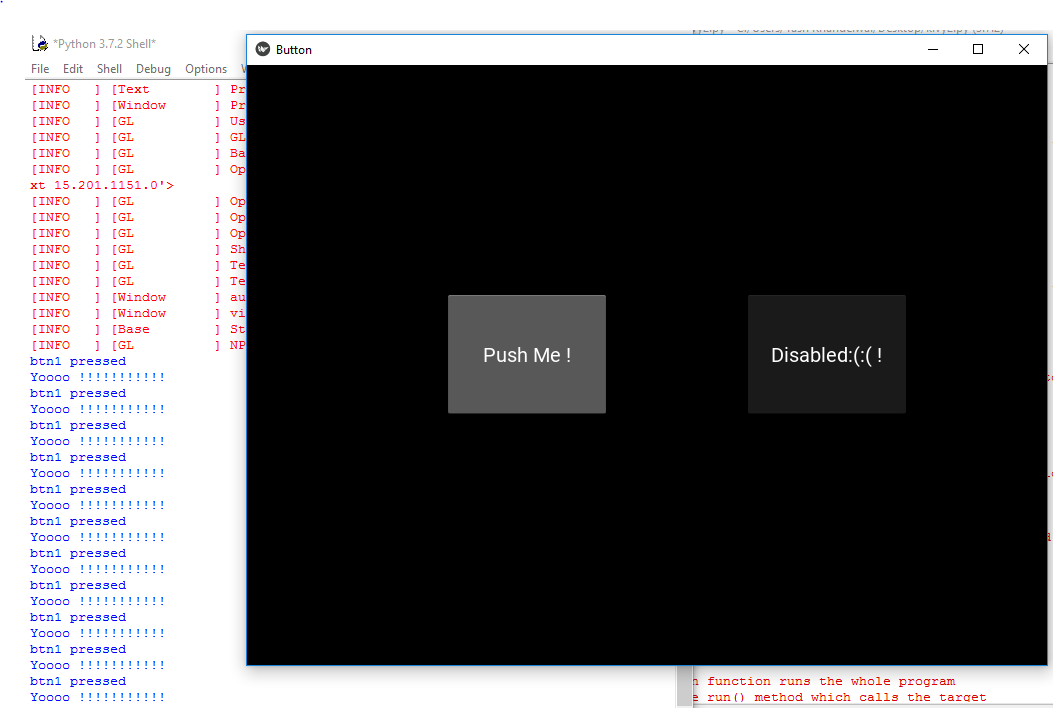# Disable Kivy Button

• Last Updated : 19 Feb, 2020

In this article, we will learn how to disable a button in kivy, there are some places where we need to disable the buttons So in this article you will learn how to do that.

Kivy Tutorial – Learn Kivy with Examples.

The Button is a Label with associated actions that are triggered when the button is pressed (or released after a click/touch). We can add functions behind the button and style the button.But to disable the button we have a property name :

`disabled that must be true`

this property will help in disabling the button i.e. button will be there but is of no use as it is disabled no functionality of button will work.

Note: disabled property was introduced in version 1.8.0. If you want to use it you need to actualize your framework.

Basic Approach to follow while creating and disabling button :

-> import kivy
-> import kivy App
-> import button
-> set minimum version(optional)
-> Extend the class
-> Add and return a button
-> Add disabled = true to disable button
-> Run an instance of the class

Firstly, let’s see how to create a fully working button and then see how to disable it and its functionality.

Code #1: How to create fully working button

 `def` `build(``self``): ` `    ``# use a (r, g, b, a) tuple ` `    ``btn ``=` `Button(text ``=``"Push Me !"``, ` `                 ``font_size ``=``"20sp"``, ` `                 ``background_color ``=``(``1``, ``1``, ``1``, ``1``), ` `                 ``color ``=``(``1``, ``1``, ``1``, ``1``), ` `                 ``size ``=``(``32``, ``32``), ` `                 ``size_hint ``=``(.``2``, .``2``), ` `                 ``pos ``=``(``300``, ``250``)) ` ` `  `    ``return` `btn `

Output:Code #2: How to disable the button

 `def` `build(``self``): ` `        ``# use a (r, g, b, a) tuple ` `        ``btn ``=` `Button(text ``=``"Push Me !"``, ` `                     ``font_size ``=``"20sp"``, ` `                     ``background_color ``=``(``1``, ``4``, ``6``, ``1``), ` `                     ``color ``=``(``1``, ``1``, ``1``, ``1``), ` `                     ``size ``=``(``32``, ``32``), ` `                     ``size_hint ``=``(.``2``, .``2``), ` `                     ``pos ``=``(``300``, ``250``), ` ` `  `                     ``# Disabling the button    ` `                     ``disabled ``=` `True` ` `  `                     ``) `

Output:Code #3: Both disable and working button together

 `# import kivy module  ` `import` `kivy  ` `   `  `# this restrict the kivy version i.e  ` `# below this kivy version you cannot  ` `# use the app or software  ` `kivy.require(``"1.9.1"``)  ` `   `  `# base Class of your App inherits from the App class.  ` `# app:always refers to the instance of your application  ` `from` `kivy.app ``import` `App  ` `   `  `# creates the button in kivy  ` `# if not imported shows the error  ` `from` `kivy.uix.button ``import` `Button  ` ` `  `# This layout allows you to set relative coordinates for children.  ` `from` `kivy.uix.relativelayout ``import` `RelativeLayout  ` ` `  `# class in which we are creating the button  ` `class` `ButtonApp(App):  ` `       `  `    ``def` `build(``self``): ` ` `  `        ``r1 ``=` `RelativeLayout() ` ` `  `         `  `        ``# working button ` `        ``btn1 ``=` `Button(text ``=``"Push Me !"``,  ` `                   ``font_size ``=``"20sp"``,  ` `                   ``background_color ``=``(``1``, ``1``, ``1``, ``1``),  ` `                   ``color ``=``(``1``, ``1``, ``1``, ``1``),  ` `                   ``size ``=``(``32``, ``32``),  ` `                   ``size_hint ``=``(.``2``, .``2``),  ` `                   ``pos ``=``(``200``, ``250``))  ` ` `  `        ``# disabled button ` `        ``btn2 ``=` `Button(text ``=``"Disabled:(:( !"``,  ` `                   ``font_size ``=``"20sp"``,  ` `                   ``background_color ``=``(``1``, ``1``, ``1``, ``1``),  ` `                   ``color ``=``(``1``, ``1``, ``1``, ``1``),  ` `                   ``size ``=``(``32``, ``32``),  ` `                   ``size_hint ``=``(.``2``, .``2``),  ` `                   ``pos ``=``(``500``, ``250``), ` ` `  `                   ``# Add disabled property true to disabled button ` `                   ``disabled ``=` `True``) ` ` `  `        ``r1.add_widget(btn1) ` `        ``r1.add_widget(btn2) ` `        `  `        ``# bind() use to bind the button to function callback  ` `        ``btn1.bind(on_press ``=` `self``.callback) ` `        ``return` `r1  ` `   `  `    ``# callback function tells when button pressed  ` `    ``def` `callback(``self``, event):  ` `        ``print``(``"button pressed"``)  ` `        ``print``(``'Yoooo !!!!!!!!!!!'``)  ` `           `  `   `  `# creating the object root for ButtonApp() class   ` `root ``=` `ButtonApp()  ` ` `  `# run function runs the whole program  ` `# i.e run() method which calls the target  ` `# function passed to the constructor.  ` `root.run()  `

Output:My Personal Notes arrow_drop_up
Recommended Articles
Page :